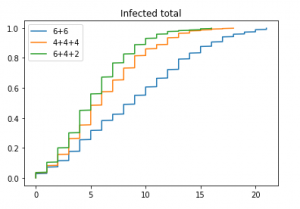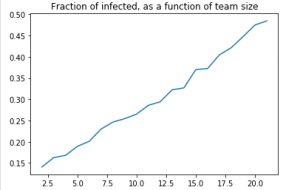## Introduction

As Covid-19 takes over the country, many organizations move their teams to work from home. Often, it is necessary to keep an office presence. This can be done in various ways: split your team into smaller units and let them alternate days, or weeks, or do completely random assignments (essentially, toss a coin for who will be in the office three days from now), etc. Or, one can abandon the fixed teams, and shuffle employees, again on a random or periodic basis…

These scenarios are apriori quite different in terms of their impact on infection propagation.  How to minimize the exposure of their personnel, is a question without an immediate answer. Below are a couple of back-of-envelope answers.

Keep in mind, that the models I look at are highly stylized, and you should always consult your physician resident mathematician (if you don’t have one, hire them) before applying them. Perhaps, the most important caveat: we consider the model where there is just one infected person interacting with the team at any given interval (so that this model might be completely irrelevant in a week or so).

To address more detailed scenarios, a simulation platform is being developed.

### Genesis of the project

A friend of a friend asked, how one can optimize rotation of the team members between office duties and work from home, to make exposure to corona virus minimal. To quote:

if they break their company into teams if it’s better to do 1) team A come in one day and team B the next or 2) randomly draw 50% for each day. Would one method over the other give them a statistical advantage over spreading corona to the team.

### TL;DR/Executive summary

We consider possible strategies to minimize impact of the presence of (latent) infected person on a team. On one hand, we look into whether it is better to randomize the number of days a person is attending the office: turns out, it is always beneficial to randomize. On the other hand, we consider whether it is desirable to keep fixed teams or shuffle people between them: turns out it is always better to keep fixed teams.

However, whichever are the general formulae, simulations still rule.

## Baseline model

Assume that one wants to reduce the chances that an infected individual in a team would infect someone else, before being diagnosed and quarantined. Assume further, for simplicity, that the latent period (while the infected person is undetected) has an even number of working days, $$2D$$, say (in practice, $$2D$$ is something like 8).
Denote the chance that the team where the infected person is present to stay coronavirus-free is $$q$$ (obviously, $$q$$ is between $$0$$ and $$1$$, but its actual value depends on the overall situation, size of the team, workplace practices etc, – and, as we will see, does not matter much in this model).

So, if the teams alternate, the chances that the team that has an infected person will see no transmission is $$q^D$$: they just have to be lucky $$D$$ times.

Consider now the teams that meet on any given day with probability $$1/2$$, over $$2D$$ days. The probability they are lucky on any given day is
$1/2+q/2$
Namely, if they don’t meet, they are fine (with probability $$= 1/2$$), and if they do, they are lucky with probability $$q$$.

So, the randomly meeting team will be lucky with probability
$(1/2 + q/2)^{2D}.$

Now the question is which is larger, $$q^D$$ or $$(1/2+q/2)^(2D)$$. This is equivalent to asking which is larger, $$q$$ or $$(1/2+q/2)^(2)$$.

Opening the brackets, we see that
$(1/2+q/2)^2-q=(1-q)^2/4>0$
for all $$q<1$$.

In other words, the randomly meeting team always fares better than on-off team

### Some more generality

What is the intuition behind this result? It is, in fact quite transparent. Think about the number of days, $$D$$, the infected person spends with the rest of the team. Given this number, the chances for his team to not get infected is $$q^D$$. The dependence of this probability on $$D$$ is convex, so that Jensen inequality kicks in, implying
$\mathbb{E} q^D\geq q^{\mathbb{E} D},$

In other words, the chances of having no transmission at all from the infected person to the rest of the team are always smaller for random D than for the deterministic D with the same mean.

Another general takeaway is that the probability of having no transmission at all does not depend on how the rest of the team is formed, – whether the team is the same on each of the $$D$$ days they interact with the infected person, or is changing.

## Random vs Fixed teams

However, if one is not just concerned with the probability of having a transmission, but also with how many people are infected, if the transmission does happen, we need more detailed analysis.

Let’s look into the average number of the team members infected, not just the probability that the number of infected people is $$0$$.

We fix (for now) the number of days $$D$$ the infected person was interacting with the rest of the team.

Assume that during each of the days, a fraction $$s$$ of the total personnel count $$N$$ is manning the office (so that $$k=sN$$ people are present each day). In the baseline model above, $$s=1/2$$.

### Two possible scenarios:

• Fixed: all k members interacting with the infected person are the same, and
• Random: each day, any person has a chance $$s$$ to be manning the office, chosen randomly each day.

Assume further, that for each person sharing the office with the infected one, the chances to avoid contracting CV is $$\tau$$ (so that the chances that all $$k$$ people present avoid contracting it on any given day is $$\tau^k=q$$).

### Fixed teams

In this case, for any of the $$k$$ people on the team, the probability to avoid transmission for $$D$$ days is $$\tau^D$$, so that the average number of people aoviding transmission is $$k\tau^D$$, and the average number of infected people is
$I_F=k(1-\tau^D).$

### Random teams

In this case, each of $$N$$ people on any given day will not be summoned to the office with probability $$(1-s)$$, and will be called in, but will escape infection with probability $$s\tau$$.

The probability to avoid infection during the $$D$$ days during which the infected person attends the office is therefore
$((1 −s) + s\tau)^D,$
and the average number of infected persons will be
$I_R=N(1 − ((1 − s) + s\tau)^D).$

### Comparison

So, who wins, $$I_F$$ or $$I_R$$? In other words, what is the comparison
$k (1 − \tau^D) \mbox{ vs }N(1 − ((1 − s) + s\tau)^D)?$
A little bit of algebra (which we will skip here) shows that
$I_F>I_R$
for any $$\tau\lt 1$$.

In other words, the number of infected persons is smaller for fixed teams, than for the randomly shuffled ones (as, perhaps, intuition was telling you anyway).

## Simulations still Rule

All these computation, general as they are, are still inferior compared to the hammer of OR and epidemiological theory: agent-based simulations. Here‘s a python notebook running such simulations, taking as an input some approximations we know from the literature (like incubation period etc), some guesstimates (like infection rates), and the compositions of the teams, and spitting various estimates, like the distribution of the number of infected.

### Example

The planning concerns a team of 24, over 60 days. The competing plans are to split them into teams of 6, working each on schedule 5 days office, 5 days at home, or into three teams, of sizes 4+4+4 or 6+4+2. THe personnel is rotated between the teams. Other parameters are:

• at work infection rate/contagious person: 0.09
• at home infection rate: 0.005

Here’s the typical output. Those are the cumulative distribution functions (say, value .5 at 5 means that the chances to have 5 or less infected is .5), so the higher the plot runs, the better the schedule is.Here’s another

### Example

We estimated the fraction of the team that will be infected over the 60 days, on the 5 days at work, 5 days at home schedule. The infection at work and infection at home rates are as above.The key feature of this graph is the the fraction increases with the size of the team. This implies, that given the number of workers and the number of teams into which you need to split your crew, it is always beneficial to keep the sizes of the teams as uniform as possible.

## Conclusion

It makes little sense to try to formulate universal recommendations that would guide staffing decisions in each particular situation. Neither is practical to attempt to work out precise formulae for a broad spectrum of scenarios. What the managers should do, is simulations, especially agent-based, – they are still the best tool in town, and can save lives.

Use them!